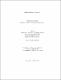2015
##### Authors
Urichuk, Andrew
University of Lethbridge. Faculty of Arts and Science
##### Publisher
Lethbridge, Alta. : University of Lethbridge, Dept. of Physics and Astronomy
##### Abstract
Fusion dimensions are integer-valued quantities equal to the dimensions of the spaces of conformal blocks, which describe the interactions of a conformal field theory (CFT). Our focus was on the Wess-Zumino-Witten models, a particularly interesting type of CFT, whose primary fields correspond to representations of affine Lie groups. Arguably, affine fusion tadpoles are the simplest g>0 fusion dimension, having only a single incoming field and g=1. We study the symmetries of the SU(N) tadpole and Verlinde formula with the intention of finding a non-negative-integer decomposition. Such a decomposition might be indicative of a combinatorial atom for fusion. From produced tables we found that tadpole values appeared to be polynomial in the level k. Several conjectures were made and we sketch a method obtaining general forms of SU(N) tadpoles via dominant weight sums.
##### Keywords
physics , Verlinde formula , conformal blocks , combinatorial , combinatorics , tadpole , affine , conformal field theory (CFT) , fusion product , Wess-Zumino-Witten (WZW) models , group , partial sums , Weyl , Galois , tensor product